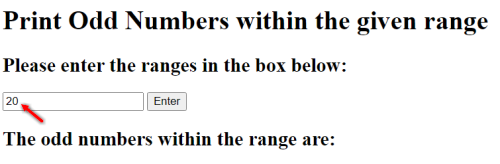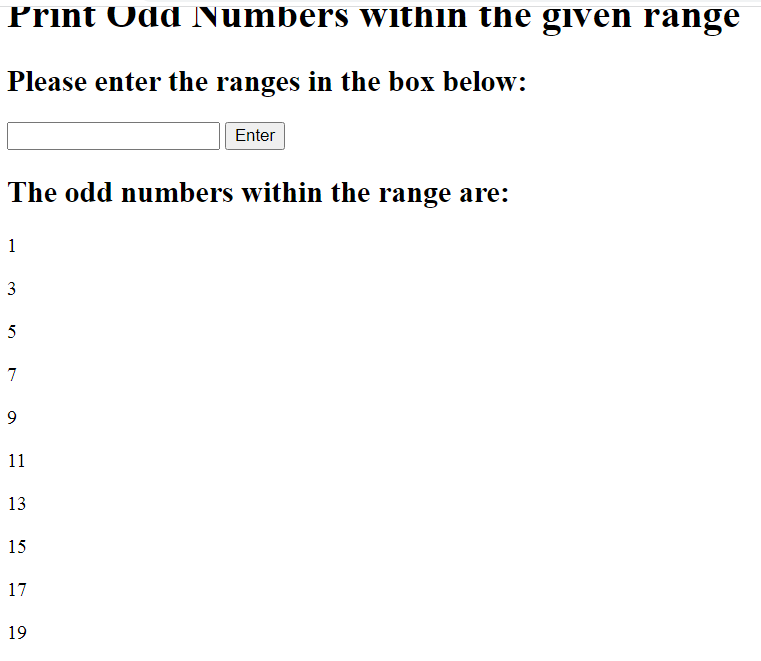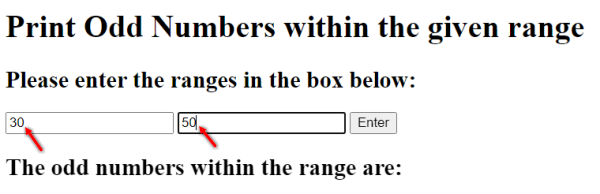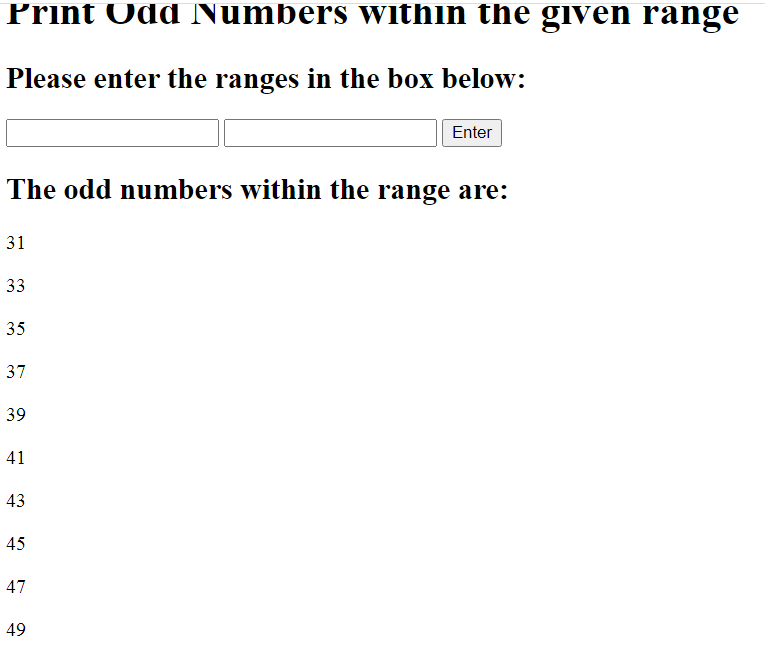# How to print Odd Numbers in JavaScript – Multiple methods

In this tutorial, let’s look at the different ways to print odd numbers in JavaScript. We’ll be using the ‘modulus’ operator to figure out whether a number is an odd number or not.

We’ll be looking at different problem statements (printing odd numbers within a range, within a user given range and within an array) and solving them one by one.

So, shall we start?

## Print odd numbers within a range

In this example, we’ll find out the list of odd numbers within a given linear range, and print them out in our browser.

To start with, let’s create our HTML skeleton. As you can see in the below code, we have our headings, and then, we have a single input box that asks the user for the range. This would be the last number in the range.

For example, if the user specifies 100, then we’ll parse the numbers from 1 to 100 and look for odd numbers in them. We have a button of id ‘enter’ right after the input box. When we create our code logic, we’ll be creating an event listener that listens to clicks of this button.

So, we’re asking the user to enter the range in the input box, and click the button right after to send the data to the ‘system’.

```<h1>Print Odd Numbers within the given range</h1>
<h2>Please enter the range in the box below:</h2>
<input type="text" id="range" />
<button id="enter">Enter</button>
```

Then, we’ll create an empty div element of id ‘nums’ and leave it as it is for now. We’ll later populate this div element with child elements (paragraphs) that contain each of the odd numbers we extract from the range.

```<h2>The odd numbers within the range are:</h2>
<div id="nums"></div>
```

I’m not going to bother with CSS styles for these examples, since the focus is on JavaScript, and its various string and number related functions. So, you can go ahead and add any styles you’d like to this mini project.

Now, let’s get the elements we need with getElementById. We need the ‘range’ input box, the ‘enter’ button and the ‘nums’ div element.

```let range = document.getElementById('range');
let enter = document.getElementById('enter');
let nums = document.getElementById('nums');
```

Then, let’s create a variable r, that’s been assigned 0 to start with. Later down the line, we’ll assign the range value inputted in the input box to this variable.

We’ve also created an empty array, oddNums, and we’ll insert the odd numbers we extract in this array, so we can later print them out.

```let r = 0;
let oddNums = Array();
```

Next, let’s create a click event listener for the ‘enter’ button, and within the anonymous function, let’s create our code logic.

To start with, we need to reset everything in case the user is entering the range for the 2nd time or more. So, if the oddNums array already has elements in it, let’s reset it back into an empty array.

And, let’s check if the ‘nums’ div element has child nodes, which means that it printed some odd numbers in the last round. Let’s use the removeChild method and remove the firstChild of nums until the nums.hasChildNodes() condition returns false (meaning, it has no child nodes left, just like it had at the start).

```enter.addEventListener('click', () => {
if(oddNums.length > 0) {
oddNums = []; //reset the array
//remove the para elements in html
while (nums.hasChildNodes()) {
nums.removeChild(nums.firstChild);
}
}
```

Now that the oddNums array and nums are empty (or the above lines of code were never traversed because the user is using the application for the first time) we can get the range that was entered in the input box and populate the oddNums array with the odd numbers within that range.

To do that, we need to parse the input inside the input box as it would be a string by default. Use the parseInt method and get the value inside the ‘range’ by using the range.value property. The 10 indicates that we need a decimal number output, which has a base of 10. Let’s assign this value to the variable ‘r’ we created at the start.

Then, let’s create a variable j, and assign it a value 0. This will contain the indices of the oddNums array.

Finally, let’s parse through the range, from 1 until r.

To find if a number is an odd number or not, we need to find the modulus of that number with 2.

For example, the result of 3 % 2 is 1, but the result of 4 % 2 is 0, and that’s how it would be for every odd and even number.
So, if a modulus of a number with 2 results in 1 (or not 0), then we have an odd number in our hands. If it results in 0, we’ve found an even number.

Using this logic, let’s figure out if the number in that specific iteration of the ‘for’ loop is an odd number. If it is, let’s add it to oddNums[j] and increment the value of j.

```    r = parseInt(range.value,10);
let j = 0;
for(let i=1; i<=r; i++) {
if(i%2 != 0) {
oddNums[j] = i;
j++;
}
}
```

Finally, let’s print the numbers.

Now, our oddNums array is populated with the odd numbers within the given range. Let’s print that out in our div element.

We’ll be using the forEach loop to iterate through the array.

For every iteration of the array, lets’ create an anonymous function where ‘o’ is the array element of that particular iteration.

Let’s create a new element p, which is of type ‘p’, meaning a HTML paragraph, and assign the innerText of p as the array element ‘o’.

Then, let’s use the appendChild method to append this ‘p’ element to the ‘nums’ div element. It’ll be appended at the end of the div element.

Once the iteration is over, make the value of the range element an empty string so the user can enter a new range and start over, if they want to.

```    //print the numbers
oddNums.forEach((o) => {
let p = document.createElement('p');
p.innerText = o;
nums.appendChild(p);
})
range.value = '';
});
```

Whew! That was a big example, but well worth it I believe, since we learned not just about finding out if a number is an odd number, but also a lot about string and array parsing, events, creating HTML elements from JavaScript etc.

If we run the above lines of code, we should get the following:I’ve entered the range as 20 and pressed enter. Then:There you go! A complete list of odd numbers from 1 to 20, as we wanted.

Here’s the entire JavaScript code for your reference.

```let range = document.getElementById('range');
let enter = document.getElementById('enter');
let nums = document.getElementById('nums');

let r = 0;
let oddNums = Array();

if(oddNums.length > 0) {
oddNums = []; //reset the array
//remove the para elements in html
while (nums.hasChildNodes()) {
nums.removeChild(nums.firstChild);
}
}
r = parseInt(range.value,10);
let j = 0;
for(let i=1; i<=r; i++) {
if(i%2 != 0) {
oddNums[j] = i;
j++;
}
}
//print the numbers
oddNums.forEach((o) => {
let p = document.createElement('p');
p.innerText = o;
nums.appendChild(p);
})
range.value = '';
});
```

## Print odd numbers within user given range

Now, let’s print odd numbers from a user given range, as in, the user gives the starting and the ending range of the calculation.

I’m not going to go into detail for this example, since we already covered a lot of what you need to know in the last example, so let me quickly explain the differences in solving this particular example.

Unlike the last example, we need 2 input boxes instead of one, and we’ll name them ‘range1’ and ‘range2’.

```    <input type="text" id="range1" />
<input type="text" id="range2" />
```

Then, let’s get all the elements, create 2 variables r1 and r1 to contain the range.

Within the click event listener code, we’ll start by checking if oddNums is already populated, and if it is, we’ll refresh both the array and the ‘nums’ div element.

Then, let’s parse the values in the 2 input boxes, get their equivalent decimal values and put them in r1 and r2.

Let’s create a for loop that starts at r1 and runs till r2, and find the odd numbers within this range.

Finally, let’s print the odd numbers by creating new paragraph elements within our main ‘div’ element and reset the values of the range1 and range2 input boxes.

```let range1 = document.getElementById('range1');
let range2 = document.getElementById('range2');
let enter = document.getElementById('enter');
let r1 = 0, r2 = 0;
let oddNums = Array();
let nums = document.getElementById('nums');

if(oddNums.length > 0) {
oddNums = []; //reset the array
//remove the para elements in html
while (nums.hasChildNodes()) {
nums.removeChild(nums.firstChild);
}
}
r1 = parseInt(range1.value,10);
r2 = parseInt(range2.value,10);
let j = 0;
for(let i=r1; i<=r2; i++) {
if(i%2 != 0) {
oddNums[j] = i;
j++;
}
}
//print the numbers
oddNums.forEach((o) => {
let p = document.createElement('p');
p.innerText = o;
nums.appendChild(p);
})
range1.value = '';
range2.value = '';
});
```

Let’s run the above lines of code and look at the output:As pointed out in the above screenshot, I’ve entered the range as 30 and 50, and when I press enter, I’ll get the following.That’s it! We’ve gotten the odd numbers from 30 to 50 printed out, as asked for.

So, there you go! We’ve looked at 2 examples to print odd numbers in JavaScript, and we created 2 mini applications while we were at it.